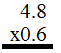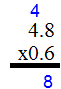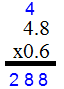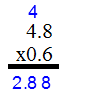### Home > MC2 > Chapter 3 > Lesson 3.1.4 > Problem3-39

3-39.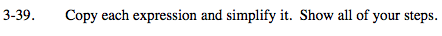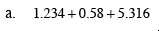Make sure you line up the decimal points.

Now add the numbers, starting from the very right and moving your way to the left.

Remember to carry over the extra 1 if a sum comes out to be 10 or higher!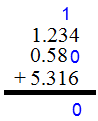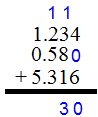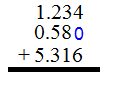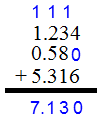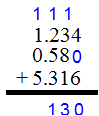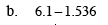Make sure you line up the decimal points.

Fill in zeroes on the top number and now you are ready to subtract. Remember to carry over a one from the left side if the number that is being subtracted exceeds the number it is being subtracted from!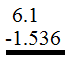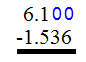4.564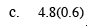When multiplying, you do NOT have to line up the decimals. But we will in this case.

Start from the left side of the equation. Remember to carry over the extra digit if the product is 10 or higher! Also remember that the extra digit is added, NOT multiplied to product.

Starting from the right count two spaces to the left. This is where the decimal should be located because the terms being multiplied both have a decimal one space from the right. These spaces added together equal two, where the new decimal point should be.